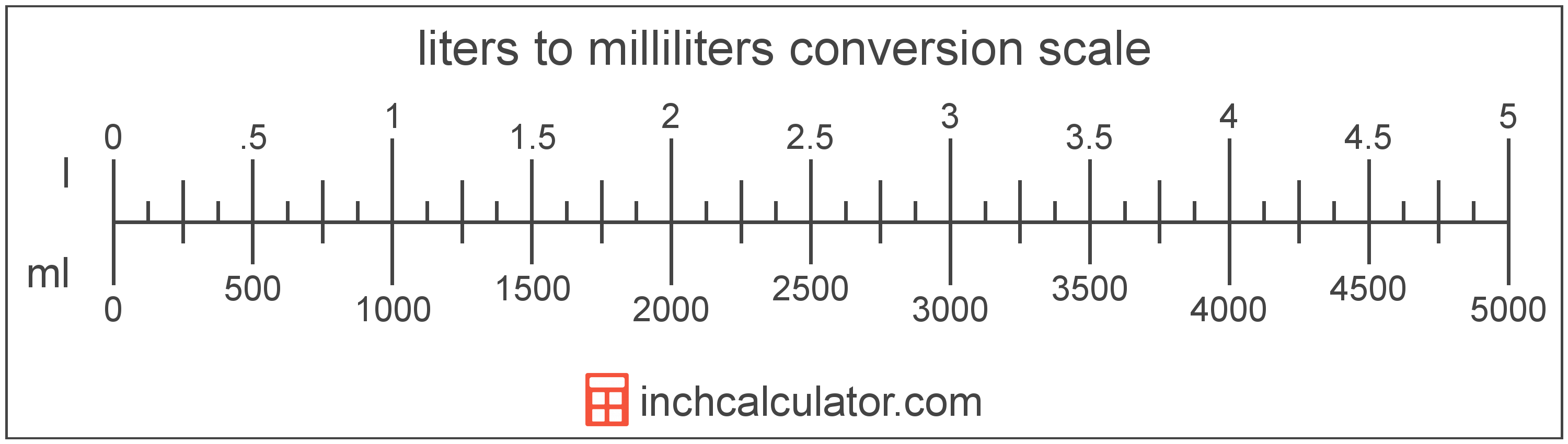# Liters to Milliliters Conversion

Enter the volume in liters below to get the value converted to milliliters.

Results in Milliliters:1 l = 1,000 ml

## How to Convert Liters to MillilitersTo convert a liter measurement to a milliliter measurement, multiply the volume by the conversion ratio.

Since one liter is equal to 1,000 milliliters, you can use this simple formula to convert:

milliliters = liters × 1,000

The volume in milliliters is equal to the liters multiplied by 1,000.

For example, here's how to convert 5 liters to milliliters using the formula above.
5 l = (5 × 1,000) = 5,000 ml

### How Many Milliliters are in a Liter?

There are 1,000 milliliters in a liter, which is why we use this value in the formula above.

1 l = 1,000 ml

## Liters

A liter is a unit of volume equal to 1,000 cubic centimeters. The liter is a special name defined for the cubic decimeter and is exactly equal to the volume of one cubic decimeter.

The liter is an SI accepted unit for volume for use with the metric system. A liter is sometimes also referred to as a litre. Liters can be abbreviated as l, and are also sometimes abbreviated as L or . For example, 1 liter can be written as 1 l, 1 L, or 1 ℓ.

## Milliliters

The milliliter is a unit of volume equal to 1 cubic centimeter, 1/1,000 of a liter, or about 0.061 cubic inches.

The milliliter is an SI unit of volume in the metric system. In the metric system, "milli" is the prefix for 10-3. A milliliter is sometimes also referred to as a millilitre. Milliliters can be abbreviated as ml, and are also sometimes abbreviated as mL or mℓ. For example, 1 milliliter can be written as 1 ml, 1 mL, or 1 mℓ.

Milliliters are sometimes expressed using the abbreviation cc for medical dosage, which is the abbreviation for the cubic centimeter.

## Liter to Milliliter Conversion Table

Liter measurements converted to milliliters
Liters Milliliters
0.001 l 1 ml
0.002 l 2 ml
0.003 l 3 ml
0.004 l 4 ml
0.005 l 5 ml
0.006 l 6 ml
0.007 l 7 ml
0.008 l 8 ml
0.009 l 9 ml
0.01 l 10 ml
0.02 l 20 ml
0.03 l 30 ml
0.04 l 40 ml
0.05 l 50 ml
0.06 l 60 ml
0.07 l 70 ml
0.08 l 80 ml
0.09 l 90 ml
0.1 l 100 ml
0.2 l 200 ml
0.3 l 300 ml
0.4 l 400 ml
0.5 l 500 ml
0.6 l 600 ml
0.7 l 700 ml
0.8 l 800 ml
0.9 l 900 ml
1 l 1,000 ml

## References

1. National Institute of Standards and Technology, Units outside the SI, https://physics.nist.gov/cuu/Units/outside.html
2. National Institute of Standards and Technology, Specifications, Tolerances, and Other Technical Requirements for Weighing and Measuring Devices, Handbook 44 - 2019 Edition, https://nvlpubs.nist.gov/nistpubs/hb/2019/NIST.HB.44-2019.pdf